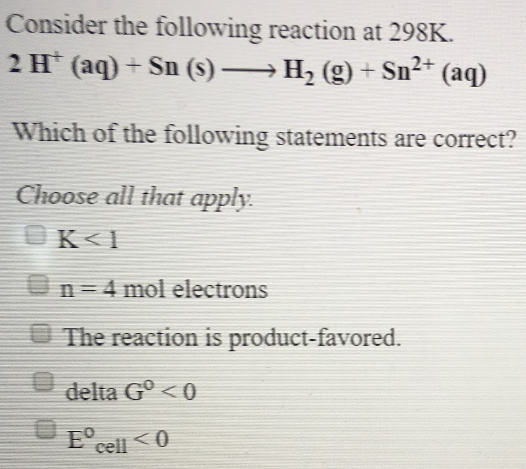# Consider the following reaction at 298K. 2 H^+ (aq) + Sn (s) → H2(g) + Sn^2+ (aq) Which of the following statements are correct? Choose all that apply. a) K < 1 b) n = 4 mol electrons c) The reaction is product-favored d) delta G ° < 0 e) E° cell < 0